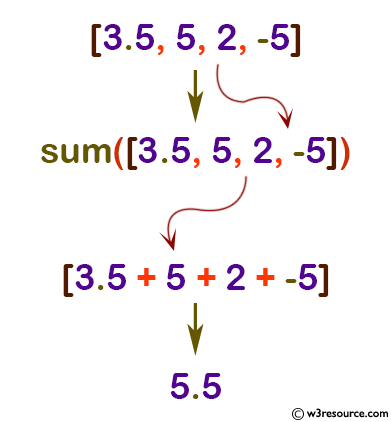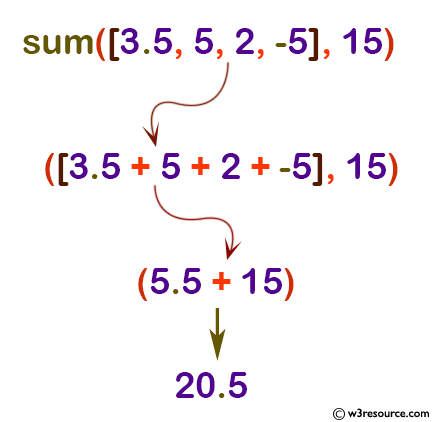# Python: sum() function

## sum() function

The sum() function is used to get the sum of all items in an iterable.

Version:

(Python 3.2.5)

Syntax:

```sum(iterable[, start])
```

Parameter:

Name Description Required /
Optional
iterable An iterable (list, tuple, dict etc) whose item's sum is to be computed. Required
start A value that is added to the return value. Optional

Return value:

The sum of the given iterable.

Example: Python sum()

``````num = [3.5, 5, 2, -5]

# start parameter is not provided
numSum = sum(num)
print(numSum)

# start = 15
numSum = sum(num, 15)
print(numSum)
``````

Output:

```5.5
20.5
```

Pictorial Presentation:Pictorial Presentation:Python Code Editor:

Previous: str()
Next: tuple()

Test your Python skills with w3resource's quiz

﻿

## Python: Tips of the Day

How do I check if a list is empty?

For example, if passed the following:

```a = []
if not a:
print("List is empty")
```

Ref: https://bit.ly/2A4JXx9